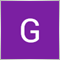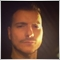# Probably a very basic question but I cant seem to find an answer, how can I get historical pricing data from MQL4 (not downloading it and reading it from a file)?29

Ideally, I would like to end up with an array like

`[(O,H,L,C)0, (O,H,L,C)1, (O,H,L,C)2 ... (O,H,L,C)n]`

where every tuple represents a candle and n=periods, n defined by me27521

1. How To Ask Questions The Smart Way. 2004
Use meaningful, specific subject headers. Not a run on, irrevalent “probably a very basic question but I cant seem to find an answer.”

2. Cant (SIC) seem to find an answer? Perhaps you should read the manual. Timeseries and Indicators Access
How To Ask Questions The Smart Way. 2004
RTFM and STFW: How To Tell You've Seriously Screwed Up.27940

Guillermo Bondonno:

Ideally, I would like to end up with an array like

where every tuple represents a candle and n=periods, n defined by me

there are 3 ways basically

B is used for the native chart only (the current symbol and timeframe) ,A and C are used for any symbol and timeframe but ,after learning how to use them you must be

careful with data requests and loading . Its an uphill battle ,expecially in indicators . For now :

```#property version   "1.00"
#property strict
//+------------------------------------------------------------------+
//| Expert initialization function                                   |
//+------------------------------------------------------------------+
int OnInit()
{
//--- create timer
//a.MQL RATES COLLECTION
MqlRates NonSeriesArray[];// element zero is the oldest ,element ArraySize()-1 is the newest
int digits=(int)SymbolInfoInteger(_Symbol,SYMBOL_DIGITS);
double usage_example=0;
//Fill Non Series
int d=CopyRates(_Symbol,_Period,0,20,NonSeriesArray);
usage_example=NonSeriesArray.high+NonSeriesArray.low+NonSeriesArray.close+NonSeriesArray.open;
//mewest element dont use it like this though
usage_example=NonSeriesArray[ArraySize(NonSeriesArray)-1].high;
//prefer this
int ne=ArraySize(NonSeriesArray)-1;
if(ne>=0){usage_example=NonSeriesArray[ne].high;}
Print("----- NON SERIES DATA -----");
PrintRates(NonSeriesArray);
//Fill Series
MqlRates SeriesArray[];//element zero is the newest ,element ArraySize()-1 is the oldest
ArraySetAsSeries(SeriesArray,true);
d=CopyRates(_Symbol,_Period,0,20,SeriesArray);
usage_example=SeriesArray.high+SeriesArray.low+SeriesArray.close+SeriesArray.open;
Print("----- SERIES DATA -----");
PrintRates(SeriesArray);
//b.Native chart data
usage_example=High+Low+Close+Open;//0 is the newest bar Bars-1 is the oldest bar
//c.Non Native chart data
usage_example=iHigh(_Symbol,_Period,0)+iLow(_Symbol,_Period,0)+iClose(_Symbol,_Period,0)+iOpen(_Symbol,_Period,0);
//---
return(INIT_SUCCEEDED);
}
void PrintRates(MqlRates &Array[])
{
for(int p=0;p<ArraySize(Array);p++){
//nothing important just illustrating access and printing times to show direction
double mid=(Array[p].open+Array[p].high+Array[p].low+Array[p].close)/4;
Print("Array["+IntegerToString(p)+"]["+TimeToString(Array[p].time,TIME_DATE|TIME_MINUTES)+"]");
}
}```